# Summary: Machine Learning 1• This + 400k other summaries
• A unique study and practice tool
• Never study anything twice again
• Get the grades you hope for
• 100% sure, 100% understanding
Remember faster, study better. Scientifically proven.## Read the summary and the most important questions on Machine Learning 1

• ### Lecture 1

This is a preview. There are 29 more flashcards available for chapter 05/09/2016

• #### What is is Machine Learning?

Algorithms for inferring unknowns from knowns.
• #### What are examples for Machine Learning applications?

House price prediction on basis of its location.
Spam filter on basis of word usage.
Handwriting/digit/speech/image recognition.
Stock price prediction on basis of historic time series.
Netflix.
Risk for getting cancer or diabetes on clinical data.
Predict protein structure of function on basis of gene location.
Credit card fraud detection.
Search engines.
Sentiment analysis based on written text.
Classifying DNA sequences based on similarities.
Automated Internet Poker Playing.
• #### Examples unsupervised learning:

Classifying handwritten digits without knowing the labels.
Credit card fraud.
representation and preprocessing of data (dimensionality reduction).
• #### What is semi-supervised learning? (not in this course)

We have datapoints, we known some of the correct datapoints but not all. You have to combine supervised and unsuperficed.
• #### What is reinforcement learning? (not in this course)

dynamical evironment and machine needs to evaluate actions given the situation to make the decisions. Partly known, partly not (see slides). In a frequentist interpretation the probability of an event is the fraction of times that event occur.
Bayesian is ofther interpretes as a quantification of plausability or the stregth of the belief for an event.
Kind of the same, bayesian is probably best.
• #### What is a random variable?

A random variable X is a stochastic variable taken from a given set of possible values/outcomes X. (dice, coin, gravitational constant g)
We will write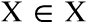but we need to distinguish between the variable and an instance/outcome x.
It might represent a stochastic "experiment" or the gathering of data.
Every random variable can be assigned a probability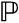(either frequency or plausibility of the outcome).

If there is an event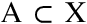we write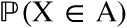for saying:
"The probability that the value of X will lie in A."
• #### What are discrete random variables?

When X is countable/finite set.

For a discrete random variablewe also write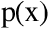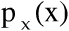or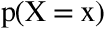for the probability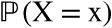is called the (probability) mass function for X.

For eventswe then have: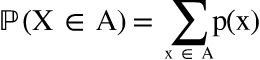meaning that the probability that X takes values in A is the sum of all probabilities that X equals x for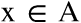.
• #### What are continuous random variables?

When X is a vector or scalar of real numbers.

Usually assume a density function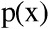. For eventwe have: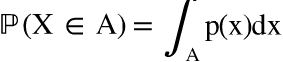Which means: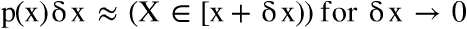• #### What is joint distribution?

It gives the probability that each of the X, Y, ... fall within a particular range or discrete set of variables specified for that variable. For example, when a coin is tossed twice: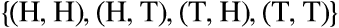• #### What is a marginal probability?

The probability distribution of the variables contained in the subset, for each of the variables, without saying something about other variables, which contrasts with conditional distribution.
To find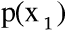all probabilities for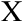have to be summed.